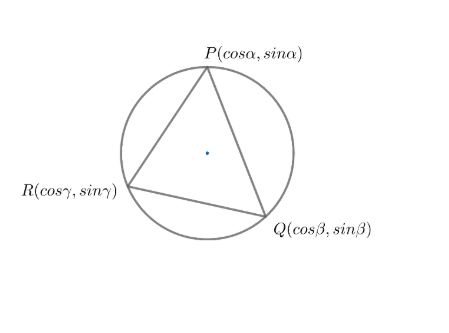Courses
Courses for Kids
Free study material
Free LIVE classes
MoreLIVE
Join Vedantu’s FREE Mastercalss

# A triangle having vertices as $P\left( \cos \alpha ,\sin \alpha \right),Q\left( \cos \beta ,\sin \beta \right),R\left( \cos \gamma ,\sin \gamma \right)$, whose orthocentre is $\left( 0,0 \right)$. Then, the value of $\cos \left( \alpha -\beta \right)+\cos \left( \beta -\gamma \right)+\cos \left( \gamma -\alpha \right)$ isA. $\dfrac{-3}{2}$ B. $\dfrac{-1}{2}$C. $\dfrac{1}{2}$D. $\dfrac{3}{2}$Verified
363.6k+ views
Hint: The centroid of the triangle divides the line joining orthocentre and the circumcentre in the ratio $2:1$.

The given vertices of the triangle are $P(\cos \alpha ,\sin \alpha ),Q\left( \cos \beta ,\sin \beta \right)$ and $R\left( \cos \gamma ,\sin \gamma \right)$.Now, we will consider the circumcircle of the triangle.
To find the equation of the circumcircle , we will see the vertices of the triangle.
By observation , we can conclude that all the vertices of the triangle satisfy the equation ${{x}^{2}}+{{y}^{2}}=1$
So , the equation of the circumcircle will be ${{x}^{2}}+{{y}^{2}}=1......(i)$
Now , we know the general equation of circle with centre at $(a,b)$ and radius $r$ is given as
${{(x-a)}^{2}}+{{(y-b)}^{2}}={{r}^{2}}$
Compared with equation$(i)$, we can say that the centre of the circle represented by equation $(i)$ is $(0,0)$ and radius is $1$ units.
So, the centre of the circumcircle is $(0,0)$ and hence the circumcentre is $(0,0)$.
Now, we know the centroid of the triangle divides the line joining orthocentre and the circumcentre in the ratio $2:1$.
Now, in the question it is given that the orthocentre of $\vartriangle ABC$ is $(0,0)$ and from equation$(i)$ we get that the circumcentre is also $(0,0)$.
So , centroid is also $(0,0)$.
We know , if a triangle has vertices $\left( {{x}_{1}},{{y}_{1}} \right),\left( {{x}_{2}},{{y}_{2}} \right)$and $\left( {{x}_{3}},{{y}_{3}} \right)$ then , its centroid is given as
$\left( \left( \dfrac{{{x}_{1}}+{{x}_{2}}+{{x}_{3}}}{3} \right),\left( \dfrac{{{y}_{1}}+{{y}_{2}}+{{y}_{3}}}{3} \right) \right)$
So , $\left( \left( \dfrac{\cos \alpha +\cos \beta +\cos \gamma }{3} \right),\left( \dfrac{\sin \alpha +\sin \beta +\sin \gamma }{3} \right) \right)=\left( 0,0 \right)$
Now,
\begin{align} & \dfrac{\cos \alpha +\cos \beta +\cos \gamma }{3}=0 \\ & \Rightarrow \cos \alpha +\cos \beta +\cos \gamma =0 \\ \end{align}
$\Rightarrow \cos \alpha +\cos \beta =-\cos \gamma$
Now , we will square both sides .
On squaring on both the sides, we get
$\Rightarrow {{\cos }^{2}}\alpha +{{\cos }^{2}}\beta +2\cos \alpha \cos \beta ={{\cos }^{2}}\gamma ......(ii)$
Again , we have $\dfrac{\sin \alpha +\sin \beta +\sin \gamma }{3}=0$
$\Rightarrow \sin \alpha +\sin \beta +\sin \gamma =0$
$\Rightarrow \sin \alpha +\sin \beta =-\sin \gamma$
Now , we will square both sides .
On squaring on both the sides, we get
$\Rightarrow {{\sin }^{2}}\alpha +{{\sin }^{2}}\beta +2\sin \alpha \sin \beta ={{\sin }^{2}}\gamma .......(iii)$
Adding $(ii)$and $(iii)$ we get ,$\left( {{\cos }^{2}}\alpha +{{\sin }^{2}}\alpha \right)+\left( {{\cos }^{2}}\beta +{{\sin }^{2}}\beta \right)+2\left( \cos \alpha \cos \beta +\sin \alpha \sin \beta \right)=\left( {{\cos }^{2}}\gamma +{{\sin }^{2}}\gamma \right)$
We know , ${{\cos }^{2}}\theta +{{\sin }^{2}}\theta =1$ and $\cos A\cos B+\sin A\sin B=\cos (A-B)$ .
So , $\left( {{\cos }^{2}}\alpha +{{\sin }^{2}}\alpha \right)+\left( {{\cos }^{2}}\beta +{{\sin }^{2}}\beta \right)+2\left( \cos \alpha \cos \beta +\sin \alpha \sin \beta \right)=\left( {{\cos }^{2}}\gamma +{{\sin }^{2}}\gamma \right)$can be written as $1+1+2\left( \cos \left( \alpha -\beta \right) \right)=1$
$\Rightarrow 2\cos \left( \alpha -\beta \right)=-1$
$\Rightarrow \cos \left( \alpha -\beta \right)=\dfrac{-1}{2}........(iv)$
Now , by symmetry we can say that
$\cos \left( \beta -\gamma \right)=\dfrac{-1}{2}......(v)$
And $\cos \left( \gamma -\alpha \right)=\dfrac{-1}{2}.......(vi)$
Adding $(iv),(v)$and $(vi)$, we get
\begin{align} & \cos \left( \alpha -\beta \right)+\cos \left( \beta -\alpha \right)+\cos \left( \gamma -\alpha \right)=\left( \dfrac{-1}{2} \right)+\left( \dfrac{-1}{2} \right)+\left( \dfrac{-1}{2} \right) \\ & \Rightarrow \cos \left( \alpha -\beta \right)+\cos \left( \beta -\alpha \right)+\cos \left( \gamma -\alpha \right)=\dfrac{-3}{2} \\ \end{align}
So , the value of $\cos \left( \alpha -\beta \right)+\cos \left( \beta -\gamma \right)+\cos \left( \gamma -\alpha \right)$ is equal to $\dfrac{-3}{2}$.
Option (a) $\dfrac{-3}{2}$ is correct answer
Note: Always remember that the centroid divides the line joining orthocentre and circumcentre in the ratio $2:1$ and not $1:2$. Students often get confused and make mistakes.
Last updated date: 29th Sep 2023
Total views: 363.6k
Views today: 6.63k